# Fraction calculator

The calculator performs basic and advanced operations with fractions, expressions with fractions combined with integers, decimals, and mixed numbers. It also shows detailed step-by-step information about the fraction calculation procedure. Solve problems with two, three, or more fractions and numbers in one expression.

## Result:

### 2/5 + 1/4 + 1/10 = 3/4 = 0.75

Spelled result in words is three quarters.

### How do you solve fractions step by step?

1. Add: 2/5 + 1/4 = 2 · 4/5 · 4 + 1 · 5/4 · 5 = 8/20 + 5/20 = 8 + 5/20 = 13/20
For adding, subtracting, and comparing fractions, it is suitable to adjust both fractions to a common (equal, identical) denominator. The common denominator you can calculate as the least common multiple of both denominators - LCM(5, 4) = 20. In practice, it is enough to find the common denominator (not necessarily the lowest) by multiplying the denominators: 5 × 4 = 20. In the next intermediate step, the fraction result cannot be further simplified by canceling.
In words - two fifths plus one quarter = thirteen twentieths.
2. Add: the result of step No. 1 + 1/10 = 13/20 + 1/10 = 13/20 + 1 · 2/10 · 2 = 13/20 + 2/20 = 13 + 2/20 = 15/20 = 5 · 3/5 · 4 = 3/4
For adding, subtracting, and comparing fractions, it is suitable to adjust both fractions to a common (equal, identical) denominator. The common denominator you can calculate as the least common multiple of both denominators - LCM(20, 10) = 20. In practice, it is enough to find the common denominator (not necessarily the lowest) by multiplying the denominators: 20 × 10 = 200. In the next intermediate step, , cancel by a common factor of 5 gives 3/4.
In words - thirteen twentieths plus one tenth = three quarters.

#### Rules for expressions with fractions:

Fractions - use the slash “/” between the numerator and denominator, i.e., for five-hundredths, enter 5/100. If you are using mixed numbers, be sure to leave a single space between the whole and fraction part.
The slash separates the numerator (number above a fraction line) and denominator (number below).

Mixed numerals (mixed fractions or mixed numbers) write as non-zero integer separated by one space and fraction i.e., 1 2/3 (having the same sign). An example of a negative mixed fraction: -5 1/2.
Because slash is both signs for fraction line and division, we recommended use colon (:) as the operator of division fractions i.e., 1/2 : 3.

Decimals (decimal numbers) enter with a decimal point . and they are automatically converted to fractions - i.e. 1.45.

The colon : and slash / is the symbol of division. Can be used to divide mixed numbers 1 2/3 : 4 3/8 or can be used for write complex fractions i.e. 1/2 : 1/3.
An asterisk * or × is the symbol for multiplication.
Plus + is addition, minus sign - is subtraction and ()[] is mathematical parentheses.
The exponentiation/power symbol is ^ - for example: (7/8-4/5)^2 = (7/8-4/5)2

#### Examples:

subtracting fractions: 2/3 - 1/2
multiplying fractions: 7/8 * 3/9
dividing Fractions: 1/2 : 3/4
exponentiation of fraction: 3/5^3
fractional exponents: 16 ^ 1/2
adding fractions and mixed numbers: 8/5 + 6 2/7
dividing integer and fraction: 5 ÷ 1/2
complex fractions: 5/8 : 2 2/3
decimal to fraction: 0.625
Fraction to Decimal: 1/4
Fraction to Percent: 1/8 %
comparing fractions: 1/4 2/3
multiplying a fraction by a whole number: 6 * 3/4
square root of a fraction: sqrt(1/16)
reducing or simplifying the fraction (simplification) - dividing the numerator and denominator of a fraction by the same non-zero number - equivalent fraction: 4/22
expression with brackets: 1/3 * (1/2 - 3 3/8)
compound fraction: 3/4 of 5/7
fractions multiple: 2/3 of 3/5
divide to find the quotient: 3/5 ÷ 2/3

The calculator follows well-known rules for order of operations. The most common mnemonics for remembering this order of operations are:
PEMDAS - Parentheses, Exponents, Multiplication, Division, Addition, Subtraction.
BEDMAS - Brackets, Exponents, Division, Multiplication, Addition, Subtraction
BODMAS - Brackets, Of or Order, Division, Multiplication, Addition, Subtraction.
GEMDAS - Grouping Symbols - brackets (){}, Exponents, Multiplication, Division, Addition, Subtraction.
Be careful, always do multiplication and division before addition and subtraction. Some operators (+ and -) and (* and /) has the same priority and then must evaluate from left to right.

## Fractions in word problems:

• Denominator 2Denominator of a fraction is 5 and numerator is 7. Write the fraction .
• Chocolate to sweetsI have 30 basket sweets and 15 chocolate in a basket. If take 5 sweet out of the basket what is the ratio in simplest form of the chocolate to the numbers of sweet left in the basket?
• Which 3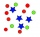Which is an equivalent ratio for 12:36
• Mass fraction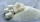2 teaspoons (24 g) of sugar are dissolved in a cup with 500 g of tea. Calculate the weight fraction of sugar in the tea.
• Double division0.25 divided by 1/2 divided by 14
• Tractors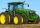6 tractors started plowing the field that they together take 12 days. Agronomist hesitates to A) after 2 days to withdraw 2 tractors, or B) after 3 days to withdraw 3 tractors. Help him which of these two cases will be plowing done sooner.
• Dinner tickets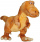At the spring festival, 7 out of the first 25 attendees had pre-purchased their dinner tickets. Based on this information, if 600 people attended the spring festival, then how many attendees could be expected to have pre-purchased their dinner tickets?
• Forest nursery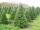In Forest nursery plant one pine to 1.9 m2. Calculate how many plants are planting in area 362 acres.
• A serving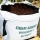A serving of rice is 1/2 of a cup. How many servings are there in a 3 1/2-cup bag of rice?
• Water in body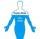Water in the human organism of all the compounds represented constitutes about 3/5 of the total weight. How many kilograms of water is in your body when you weigh 51 kg?
• Penny coinsOne 2p coin is 1/8 inch thick. A pile of 2p is 1 1/2 inch high. How many coin are there in the pileAdam has half the money in his right pocket than in his left pocket. If he transferred 40 crowns from the left pocket to the right, he would have the same in both pockets. Calculate how many crowns does Adam has in his left pocket more than in his right?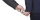Determine the salary of a part-time worker if the employer deducted (taxes etc) CZK 840 from him, which was 6/50 of his salary.